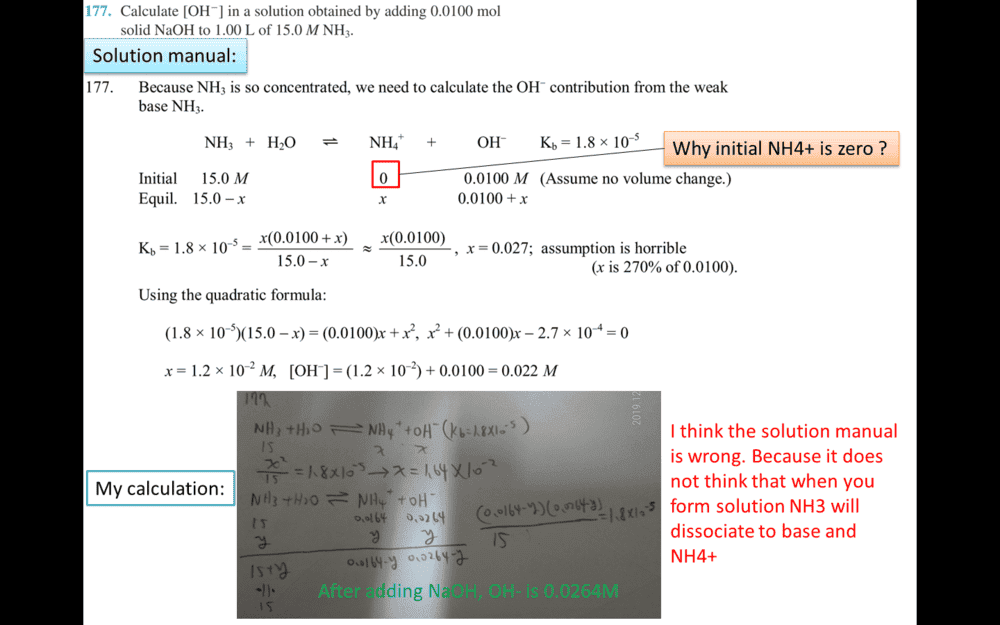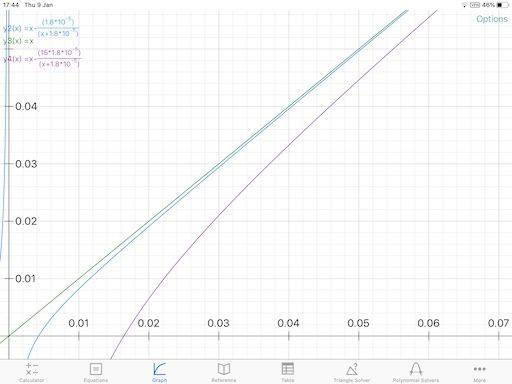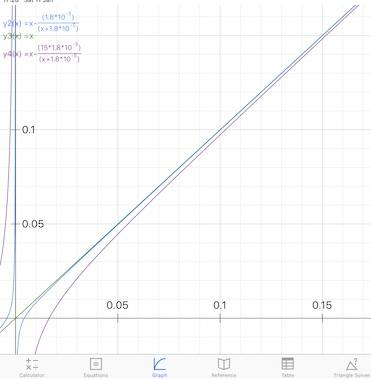# How to Make a Delicious Pie Crust

• Chemistry
• r12214001

#### r12214001

Homework Statement
Calculate [OH- ] in a solution obtained by adding 0.0100 mol
solid NaOH to 1.00 L of 15.0 M NH3 .
Relevant Equations
Is the solution manual goes wrong? Or I am confused by my self? please educate meInitial is a theoretical state before the reaction started. Note that in such cases it typically doesn't matter in what order we mix reagents, nor what were the intermediate states. In the end the equilibrium is always the same.

•Lord Jestocost
It's a good question. I could not read the student's solution but my first calculation gave me exactly the same result as his so we must have been thinking the same.

Generic thoughts that are usually valid but not quite in this case are: if you get quadratics in a problem of this kind it is because you have overlooked some simplifying approximation; or that buffering power is maximum at pH = pKa (= 9.255) while here we are far away and on the very edge where buffering power is in principle zero (the pH of 15 M ammonia solution is 12.21). But actually normal thoughts about buffering are a bit out of place here because normally we would be thinking about what happens when we add strong acid to an ammonia solution not strong base.

The precise error is we both assumed that the molarity (0.01 M) of added NaOH equal the increase of [OH-] which is not quite right in the precise conditions (high ammonia concentration, low amount of added Na+). But what is happening is that while a high concentration of ammonia solution is mostly NH3 still there is a small amount of NH4+ - 0.00424 M and 0.00164 M for 1M and 15 M total ammonia respectively*. So as strong base is added these small amounts are deprotonated particularly within the first small amounts added and the Δ[OH-] < Δ[Na+]. That is like buffering, but this is not what we normally think of as buffering, or if it is it is by buffer of very low concentration.

*(These concentrations are calculated from the approximate equation ##\left[ OH^{-}\right] =\sqrt {N_{T}K_{b}}##. A more exact calculation is solving the quadratic below for [Na+] = 0, but the two agree to the third decimal place.)

Thus solving the quadratic (which we don't really need to do, see below)
$$x^{2}+x\left( K_{b}-\left[ Na^{+}\right] \right) -K_{b}\left( N_{T}+\left[ Na^{+}\right] \right) =0$$
where NT is total concentration of the weak base and ##x## is [OH-], we find that on adding 0.01 M NaOH Δ[OH-] = 0.0072 < 0.01. This pseudobuffering soon runs out: on a second such addition Δ[OH-] = 0.0093 and on a third 0.00973, pretty close to the NaOH added. At that point pH = 12.49 by the way, everything here is happening in a small pH range. At !5 M ammonia I calculate for the first ∆[Na+] of 0.01, ∆[OH-] is as low as 0.0057, an appreciable pseudobuffering effect for the somewhat special and narrow-range conditions chosen.

I take it as understood how the quadratic equation above is derived from the equilibrium equation, a mass conservation equation and an 'electroneutrality' condition with the excellent approximation ##\left[ H^{+}\right] =0##. For understanding and even most quantitative solutions this quadratic is not that necessary nor helpful and better for understanding is its inverse

$$\left[ Na^{+}\right] =x+\dfrac {K_{b}N_{T}}{x+K_{b}}$$
Here the corresponding curves for NT = 1 (blue) and 15 M (purple).
(Vertical co-ordinate [Na+], horizontal distances between any two points represent Δ[OH-] - note that pure ammonia solution [OH-] is that where the curve for the corresponding NT intersects the abscissa.)We see that after an early deviation they soon approximate to the line [Na+] = ##x##, more slowly with the massive 15 M concentration.

Talking of which all this this is all very well for fine understanding of our equations, but I would think at 15 M ammonia the dielectric constant of the solution would have changed the given Kb quite a lot from the one given, that will have been determined in a more dilute solutoon.

#### Attachments

Last edited:
•r12214001 and etotheipi
It's a good question. I could not read the student's solution but my first calculation gave me exactly the same result as his so we must have been thinking the same.

Generic thoughts that are usually valid but not quite in this case are: if you get quadratics in a problem of this kind it is because you have overlooked some simplifying approximation; or that buffering power is maximum at pH = pKa (= 9.255) while here we are far away and on the very edge where buffering power is in principle zero (the pH of 15 M ammonia solution is 12.21). But actually normal thoughts about buffering are a bit out of place here because normally we would be thinking about what happens when we add strong acid to an ammonia solution not strong base.

The precise error is we both assumed that the molarity (0.01 M) of added NaOH equal the increase of [OH-] which is not quite right in the precise conditions (high ammonia concentration, low amount of added Na+). But what is happening is that while a high concentration of ammonia solution is mostly NH3 still there is a small amount of NH4+ - 0.00424 M and 0.00164 M for 1M and 15 M total ammonia respectively*. So as strong base is added these small amounts are deprotonated particularly within the first small amounts added and the Δ[OH-] < Δ[Na+]. That is like buffering, but this is not what we normally think of as buffering, or if it is it is by buffer of very low concentration.

*(These concentrations are calculated from the approximate equation ##\left[ OH^{-}\right] =\sqrt {N_{T}K_{b}}##. A more exact calculation is solving the quadratic below for [Na+] = 0, but the two agree to the third decimal place.)

Thus solving the quadratic (which we don't really need to do, see below)
$$x^{2}+x\left( K_{b}-\left[ Na^{+}\right] \right) -K_{b}\left( N_{T}+\left[ Na^{+}\right] \right) =0$$
where NT is total concentration of the weak base and ##x## is [OH-], we find that on adding 0.01 M NaOH Δ[OH-] = 0.0072 < 0.01. This pseudobuffering soon runs out: on a second such addition Δ[OH-] = 0.0093 and on a third 0.00973, pretty close to the NaOH added. At that point pH = 12.49 by the way, everything here is happening in a small pH range. At !5 M ammonia I calculate for the first ∆[Na+] of 0.01, ∆[OH-] is as low as 0.0057, an appreciable pseudobuffering effect for the somewhat special and narrow-range conditions chosen.

I take it as understood how the quadratic equation above is derived from the equilibrium equation, a mass conservation equation and an 'electroneutrality' condition with the excellent approximation ##\left[ H^{+}\right] =0##. For understanding and even most quantitative solutions this quadratic is not that necessary nor helpful and better for understanding is its inverse

$$\left[ Na^{+}\right] =x+\dfrac {K_{b}N_{T}}{x+K_{b}}$$
Here the corresponding curves for NT = 1 (blue) and 15 M (purple).
(Vertical co-ordinate [Na+], horizontal distances between any two points represent Δ[OH-] - note that pure ammonia solution [OH-] is that where the curve for the corresponding NT intersects the abscissa.)

View attachment 255471

View attachment 255476

We see that after an early deviation they soon approximate to the line [Na+] = ##x##, more slowly with the massive 15 M concentration.

Talking of which all this this is all very well for fine understanding of our equations, but I would think at 15 M ammonia the dielectric constant of the solution would have changed the given Kb quite a lot from the one given, that will have been determined in a more dilute solutoon.
Being surprised with tour detailed inteprenetion TKS